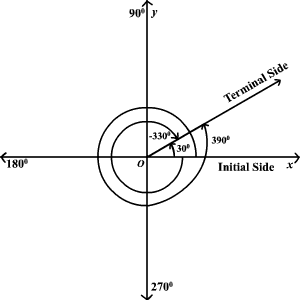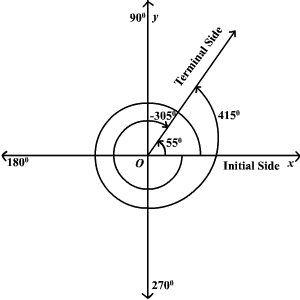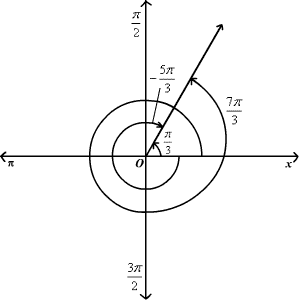# Coterminal Angles

Coterminal angles are angles in standard position (angles with the initial side on the positive $x$ -axis) that have a common terminal side.  For example $30°$ , $-330°$ and $390°$ are all coterminal.To find a positive and a negative angle coterminal with a given angle, you can add and subtract $360°$ if the angle is measured in degrees or $2\pi$ if the angle is measured in radians .

Example 1:

Find a positive and a negative angle coterminal with a $55°$ angle.

$\begin{array}{l}55°-360°=-305°\\ 55°+360°=415°\end{array}$

A $-305°$ angle and a $415°$ angle are coterminal with a $55°$ angle.Example 2:

Find a positive and a negative angle coterminal with a $\frac{\pi }{3}$ angle.

$\begin{array}{l}\frac{\pi }{3}+2\pi =\frac{7\pi }{3}\\ \frac{\pi }{3}-2\pi =-\frac{5\pi }{3}\end{array}$

A $\frac{7\pi }{3}$ angle and a $-\frac{5\pi }{3}$ angle are coterminal with a $\frac{\pi }{3}$ angle.